Viktor LevandovskyyAreas of Research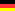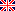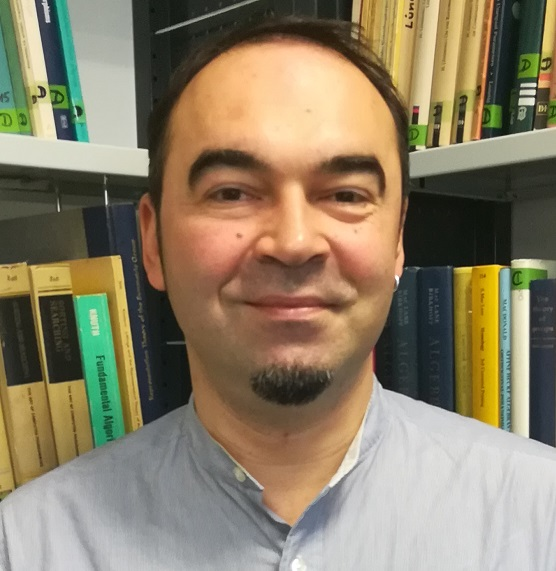Home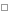CVResearch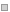Teaching(For) StudentsPublicationsProjectsConferencesMy SINGULARSongsNoncommutative computer algebraI am interested in Gröbner bases and their applications in different noncommutative structures - free and path algebras, iterated Ore extensions, algebras with PBW bases. In my research I continue the research line, initiated by Kandri-Rody, Weispfenning (algebras of solvable type), Apel (G-algebras) and Gomez-Torrecillaz, Lobillo et.al. (PBW algebras). Gröbner bases for these algebras and many important algorithms, based on them (like Gröbner basics) have been implemented in the computer algebra system SINGULAR : PLURAL . Future plans include partial Ore localizations of G-algebras. See my publications for more details.Noncommutative algebraG-algebras, introduced by Joachim Apel, appear in a variety of situations, coming from the different fields of science. I refined this class, calling G-algebra (resp. GR-algebra) direct generalization of commutative ring in n variables (resp. commutative ring or its factor ring). I discovered the necessary and sufficient conditions for a G-algebra to have a PBW basis and gave new simple proofs for e.g. noetherian and integral properties of them.One of the important algorithms, which has no analogue in the commutative algebra, is the preimage of a left module under a morphism between two non-commutative algebras. It has been designed and implemented, there are important applications of this algorithm to different problems in mathematics.Algebraic Systems and Control TheoryThe module--theoretic approach to problems of Systems and Control Theory provides a possibility to apply algorithms of Computer Algebra and of constructive Homological Algebra. In cooperation with Eva Zerz (Aachen), we study various problems, arising in Systems and Control Theory. In particular, a SINGULAR library control.lib was created. See more details on the project at Control Library In Plural and Singular (CLIPS) .Another interesting library, implemented in the framework of the project is involut.lib. The procedures of this library can compute the set of involutions (that is, involutive antiisomorphisms) of a given non-commutative polynomial algebra.We are working further on generalizing the results and algorithms to the non-commutative Ore algebras and, in general, to partial Ore localizations of G-algebras.Symbolical and Numerical ComputationWith Bernd Martin (Cottbus), we are working on symbolic methods for (systems of) linear partial differential equations. Our current computational framework consists of SINGULAR library, MATHEMATICA built-in functions and an interoperability package between MATHEMATICA and SINGULAR . The forthcoming paper describes the ideas behind this framework. In particular, one can efficiently perform the following tasks:based on given formulas for approximations, generate a finite difference scheme for an equation (a system of equations)analyze a given scheme for von Neumann stability and obtain conditions for stabilityanalyze a given stable scheme for its dispersionRepresentation Theory of AlgebrasThere are many problems in representation theory of algebras, requiring intervention from the computer algebra side. Some of them are really hard (and thus, interesting). Some of them could not be solved algorithmically (thinking in a classical sense of the "algorithm" paradigm). Here are some algorithms I am interested in:Maximal two-sided ideal in a given left ideal (for computing e.g. the annihilator of a left module)Test for the maximality of a given left ideal (equivalently, test for the simplicity of a left module)
In my dissertation (2005, available online, see publications ), there are some results on these and other topics. In particular, one is able to compute the good approximation of the annihilator of a left module for so-called holonomic modules.Together with O. Khomenko (Freiburg) we developed an algorithm for computing the Central Character Decomposition of a left module. It is implemented in the library ncdecomp.lib, distributed with SINGULAR : PLURAL since 2003.RWTH Aachen University Division of Mathematics Lehrstuhl D für Mathematik